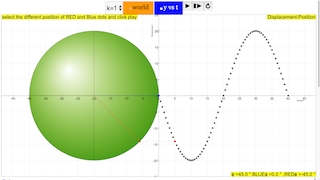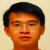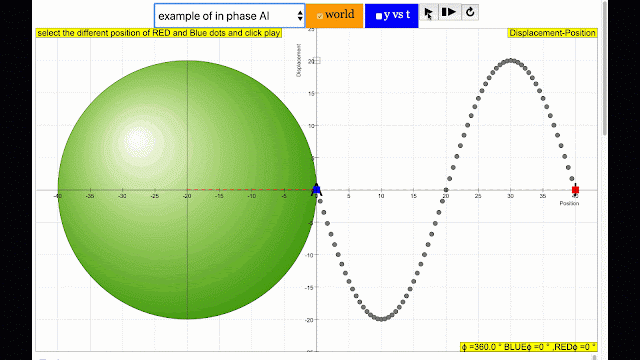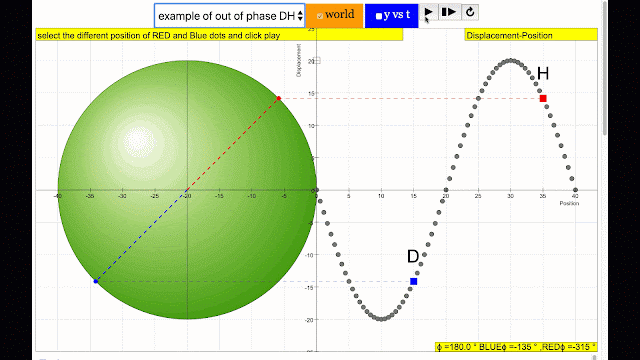Topics

Transverse and longitudinal waves

Description

In this Transverse wave model, the simulation shows the phase difference commonly associated in wave motion in terms of angle differences between the 2 points travelling in a circular motion.
Play with the Model. Test what you've learned by exploring the  phase difference, amplitudes, wavelengths, periods, frequencies and  wave velocities.

Sample Learning Goals

(a) show an understanding and use the terms displacement, amplitude, phase difference, period,
frequency, wavelength and speed
(f) analyse and interpret graphical representations of transverse and longitudinal waves

Activities

1. By changing i and j sliders, the different positions of a wave can be simulated.
2. Determine the phase difference between the BLUE and RED point using ϕ/(2π) = t/T or otherwise.
3. Use the simulation to check your calculation.
4. The slider T changes the period of the wave motion, by means of evidences from the simulation or otherwise, determine the period
5. The slider A changes the amplitude of the wave motion, by means of evidences from the simulation or otherwise, determine the amplitude
6. the guide allows the visualization of the front view of the point BLUE and RED.

Version:

Intro Page

Translations

Code Language Translator RunSoftware Requirements

SoftwareRequirements

 Android iOS Windows MacOS with best with Chrome Chrome Chrome Chrome support full-screen? Yes. Chrome/Opera No. Firefox/ Samsung Internet Not yet Yes Yes cannot work on some mobile browser that don't understand JavaScript such as..... cannot work on Internet Explorer 9 and below

CreditsLee Tat Leong; Wee Loo Kang

Description

Phase is the position of a point in time (an instant) on a waveform cycle. A complete cycle is defined as the interval required for the waveform to return to its arbitrary initial value. The graph to the right shows how one cycle constitutes 360° of phase.phase difference equal to zero also called in phase. notice point A and I are moving up and down the wave where their projected position on the circle form 0 degree difference, thus called in phase.phase difference equal to 180 degrees also called antiphase. notice point A and E are moving up and down the wave where their projected position on the circle form 180 degree difference, thus called antiphase. Note that out of phase is implied but insufficient to describe the relation as out of phase can also mean angles from 1 to 179 degrees.phase difference equal to 180 degrees also called antiphase. notice point B and F are moving up and down the wave where their projected position on the circle form 180 degree difference, thus called antiphase. Note that out of phase is implied but insufficient to describe the relation as out of phase can also mean angles from 1 to 179 degrees.phase difference equal to 180 degrees also called antiphase. notice point D and H are moving up and down the wave where their projected position on the circle form 180 degree difference, thus called antiphase. Note that out of phase is implied but insufficient to describe the relation as out of phase can also mean angles from 1 to 179 degrees.phase difference equal to 180 degrees also called antiphase. notice point E and I are moving up and down the wave where their projected position on the circle form 180 degree difference, thus called antiphase. Note that out of phase is implied but insufficient to describe the relation as out of phase can also mean angles from 1 to 179 degrees.phase difference equal to 180 degrees also called antiphase. notice point E and I are moving up and down the wave where their projected position on the circle form 180 degree difference, thus called antiphase. Note that out of phase is implied but insufficient to describe the relation as out of phase can also mean angles from 1 to 179 degrees.phase difference equal to 180 degrees also called antiphase. notice point C and G are moving up and down the wave where their projected position on the circle form 180 degree difference, thus called antiphase. Note that out of phase is implied but insufficient to describe the relation as out of phase can also mean angles from 1 to 179 degrees.